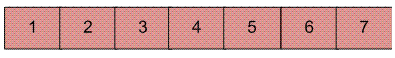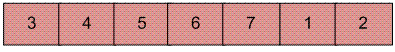Open in App
Not now

# C Program for Reversal algorithm for array rotation

• Difficulty Level : Basic
• Last Updated : 28 May, 2022

Write a function rotate(arr[], d, n) that rotates arr[] of size n by d elements.

Example:

```Input:  arr[] = [1, 2, 3, 4, 5, 6, 7]
d = 2
Output: arr[] = [3, 4, 5, 6, 7, 1, 2]
```Rotation of the above array by 2 will make array## Recommended: Please solve it on “PRACTICE ” first, before moving on to the solution.

Algorithm :

```rotate(arr[], d, n)
reverse(arr[], 1, d) ;
reverse(arr[], d + 1, n);
reverse(arr[], 1, n);
```

Let AB are the two parts of the input array where A = arr[0..d-1] and B = arr[d..n-1]. The idea of the algorithm is :

• Reverse A to get ArB, where Ar is reverse of A.
• Reverse B to get ArBr, where Br is reverse of B.
• Reverse all to get (ArBr) r = BA.

Example :
Let the array be arr[] = [1, 2, 3, 4, 5, 6, 7], d =2 and n = 7
A = [1, 2] and B = [3, 4, 5, 6, 7]

• Reverse A, we get ArB = [2, 1, 3, 4, 5, 6, 7]
• Reverse B, we get ArBr = [2, 1, 7, 6, 5, 4, 3]
• Reverse all, we get (ArBr)r = [3, 4, 5, 6, 7, 1, 2]

Below is the C implementation of the above approach :

 `// C/C++ program for reversal algorithm of array rotation``#include ` `/*Utility function to print an array */``void` `printArray(``int` `arr[], ``int` `size);` `/* Utility function to reverse arr[] from start to end */``void` `rvereseArray(``int` `arr[], ``int` `start, ``int` `end);` `/* Function to left rotate arr[] of size n by d */``void` `leftRotate(``int` `arr[], ``int` `d, ``int` `n)``{``    ``rvereseArray(arr, 0, d - 1);``    ``rvereseArray(arr, d, n - 1);``    ``rvereseArray(arr, 0, n - 1);``}` `/*UTILITY FUNCTIONS*/``/* function to print an array */``void` `printArray(``int` `arr[], ``int` `size)``{``    ``int` `i;``    ``for` `(i = 0; i < size; i++)``        ``printf``(``"%d "``, arr[i]);``}` `/*Function to reverse arr[] from index start to end*/``void` `rvereseArray(``int` `arr[], ``int` `start, ``int` `end)``{``    ``int` `temp;``    ``while` `(start < end) {``        ``temp = arr[start];``        ``arr[start] = arr[end];``        ``arr[end] = temp;``        ``start++;``        ``end--;``    ``}``}` `/* Driver program to test above functions */``int` `main()``{``    ``int` `arr[] = { 1, 2, 3, 4, 5, 6, 7 };``    ``int` `n = ``sizeof``(arr) / ``sizeof``(arr);``    ``int` `d = 2;``    ``leftRotate(arr, d, n);``    ``printArray(arr, n);``    ``return` `0;``}`

Output:

```3 4 5 6 7 1 2
```

Time Complexity : O(n)
Auxiliary Space : O(1)

Please refer complete article on Reversal algorithm for array rotation for more details!

My Personal Notes arrow_drop_up